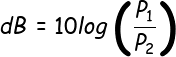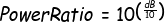# dB Calculator

Input either a dB value or power ratio and hit 'enter' ('tab' if using Internet Explorer) to convert from one to the other.

### Power Ratio:

The equation to get the dB value from a power ratio is:The equation to get the power ratio from a dB value is: